## 广汽菲克 自由光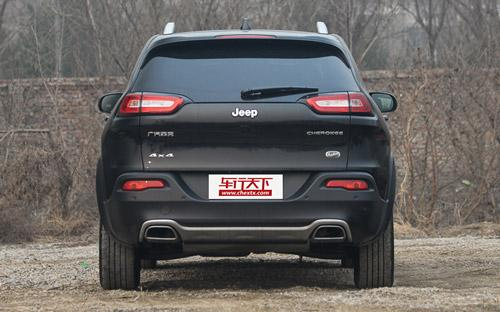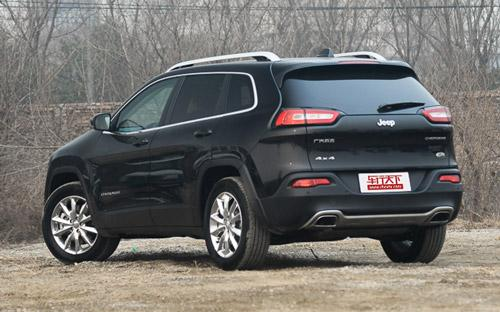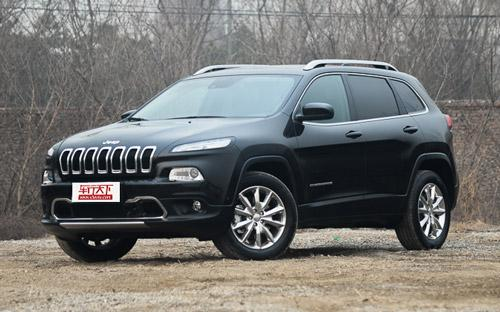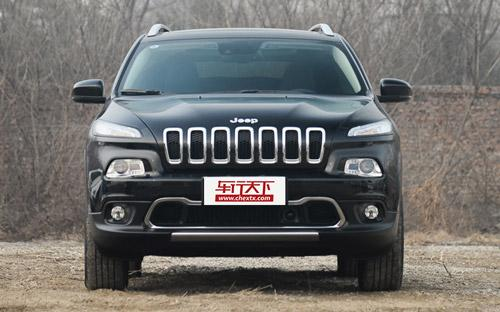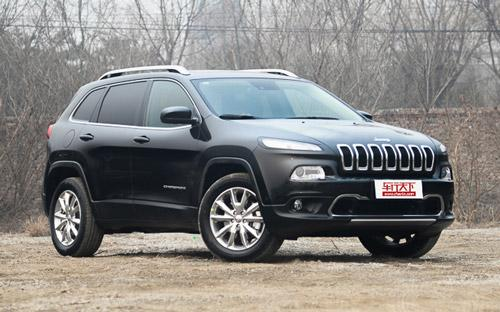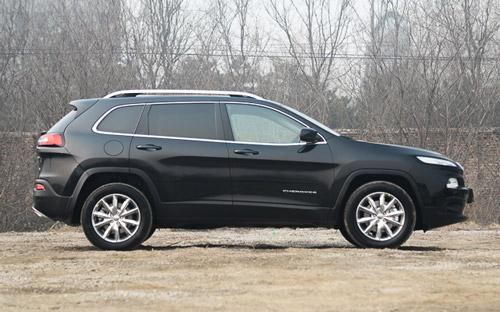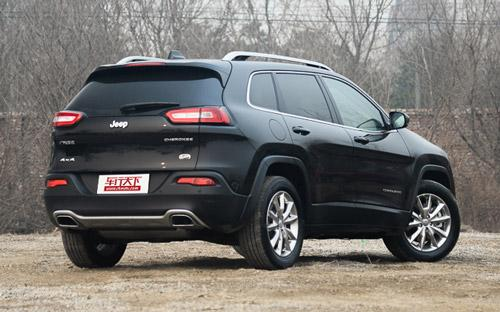9 种颜色可选2016款最低售价：22.98 万元起

4649(mm)1859(mm)1686(mm)##### 配置亮点：
• 胎压监测装置

• ISOFIX儿童座椅接口

• 车身稳定控制(ESC/ESP/DSC等)

• 电动天窗

• 定速巡航

• 后倒车雷达

• 真皮座椅

• GPS导航系统

• 氙气大灯

• 后视镜加热

2016款 2.4L 领先版 (240张)
• 2016款 2.4L 领先版 (240张)
• 2016款 2.4L 专业版智能包 (226张)广汽菲克 自由光 在售车型

排量 车型 厂商指导价 本地最低报价 购车工具
2.0L 2.4L 领先版 9挡手自一体 22.98万 22.98万

询底价+对比
2.4L 专业版 9挡手自一体 24.98万 询底价+对比
2.4L 专业版智能包 9挡手自一体 25.98万 询底价+对比
2.4L 优越版 9挡手自一体 27.58万 询底价+对比
2.4L 全能版 9挡手自一体 31.58万 31.58万

询底价+对比
2.0L 领先版 9挡手自一体 20.98万 20.98万

询底价+对比
2.0L 智能版 9挡手自一体 21.58万 询底价+对比
2.0L 优越版 9挡手自一体 21.98万 询底价+对比
2.4L 2.4L 领先版 9挡手自一体 22.98万 22.98万

询底价+对比
2.4L 专业版 9挡手自一体 24.98万 询底价+对比
2.4L 专业版智能包 9挡手自一体 25.98万 询底价+对比
2.4L 优越版 9挡手自一体 27.58万 询底价+对比
2.4L 全能版 9挡手自一体 31.58万 31.58万

询底价+对比
2.0L 领先版 9挡手自一体 20.98万 20.98万

询底价+对比
2.0L 智能版 9挡手自一体 21.58万 询底价+对比
2.0L 优越版 9挡手自一体 21.98万 询底价+对比

广汽菲克 自由光 经销商

查看更多 >>
更多 >>

本地降价排名

### 广汽菲克 自由光 动力加速

自由光 0-100公里加速时间分布在 0.0-秒 属于 超跑级

动力级别 加速时间 车型

广汽菲克 自由光 视频

广汽菲克 自由光 新闻资讯

# 清明节假期自驾游 自由光提供专业舒适的解决方案

导购 超过9149次关注

继春节后，终于迎来了首个小长假，持续快节奏的工作近一个多月后，是不是又开始计划一场放松的全家自驾游？所以，选车就很关键了，选错，会让囧途更囧。而选全新Jee...

# 外观微调 Jeep新款自由光或将于5月上市

即将上市 超过7590次关注

2017上海车展上Jeep正式发布了新款自由光车型，我们则从Jeep展台获悉，这款车有望在5月份正式上市销售。

# 国产Jeep自由光上市 售22.98-31.58万元

国产新车 超过9229次关注

广汽菲亚特克莱斯勒旗下的国产Jeep自由光今日在国内正式上市，新车仅搭载2.4L自然吸气发动机和9AT变速箱，按配置不同共推出五款车型，售价为22.98万元-31.58万元。

# 国产自由光2.0L上市 售价20.98-21.98万元

新闻 超过9144次关注

国产自由光于今年11月3日正式上市，2.0L车型的上市，将进一步降低入门门槛。此次推出的2.0L车型基本延续了此前的造型，看配备的是代号为M079的2.0L自然吸气发动机，...

# 预售20万元起 国产自由光2.0L配置信息

即将上市 超过9185次关注

我们从官方了解到了国产自由光2.0L相关配置信息，将标配整车声学包、前排双侧隔音玻璃、PM2.5车内空气净化系统、电子手刹、前排USB接口、智能无钥匙进入以及一键启...

# 明年1月上市 曝国产Jeep自由光2.0L实车

国产新车 超过8300次关注

近日，我们获得了一组广汽菲克Jeep自由光2.0L车型的实车照，整体造型基本与此前上市的2.4L车型保持一致，前脸底部格栅取消了两处镀铬装饰条，配备的是一台代号为M07...

猜你喜欢

﻿
• 快速找车
• 选择品牌
• 选择品牌
• A  奥迪
• A  阿斯顿·马丁
• A  阿尔法·罗密欧
• B  宝沃
• B  布加迪
• B  巴博斯
• B  保时捷
• B  宾利
• B  奔驰
• B  宝马
• B  本田
• B  别克
• B  标致
• B  比亚迪
• B  宝骏
• B  北汽制造
• B  北汽新能源
• B  北汽幻速
• B  北汽威旺
• B  北京汽车
• B  奔腾
• B  北汽绅宝
• C  长安
• C  长安商用
• C  长城
• C  昌河
• D  大众
• D  道奇
• D  DS
• D  东南
• D  东风风神
• D  东风风行
• D  东风小康
• D  东风风度
• D  东风
• F  福特
• F  丰田
• F  菲亚特
• F  法拉利
• F  福田
• F  福迪
• F  福汽启腾
• G  观致
• G  广汽传祺
• G  广汽吉奥
• G  GMC
• H  红旗
• H  汉腾汽车
• H  哈弗
• H  哈飞
• H  海格
• H  海马
• H  华颂
• H  黄海
• H  华泰
• H  恒天
• J  吉利汽车
• J  捷豹
• J  Jeep
• J  江淮
• J  江铃
• J  金杯
• J  九龙
• J  金旅
• K  凯翼
• K  凯迪拉克
• K  克莱斯勒
• K  科尼塞克
• K  卡威
• K  开瑞
• L  路虎
• L  林肯
• L  劳斯莱斯
• L  兰博基尼
• L  雷克萨斯
• L  铃木
• L  雷诺
• L  理念
• L  力帆
• L  莲花汽车
• L  猎豹
• L  路特斯
• L  陆风
• M  马自达
• M  MG
• M  MINI
• M  玛莎拉蒂
• M  摩根
• M  迈凯轮
• N  纳智捷
• O  欧宝
• O  讴歌
• O  欧朗
• Q  奇瑞
• Q  起亚
• Q  启辰
• R  日产
• R  荣威
• R  瑞麒
• S  三菱
• S  斯威汽车
• S  萨博
• S  smart
• S  斯柯达
• S  斯巴鲁
• S  思铭
• S  双龙
• S  上汽大通
• S  双环
• T  特斯拉
• T  腾势
• W  沃尔沃
• W  五菱汽车
• W  五十铃
• W  威兹曼
• W  威麟
• X  现代
• X  雪佛兰
• X  雪铁龙
• X  西雅特
• Y  一汽
• Y  英菲尼迪
• Y  英致
• Y  依维柯
• Y  野马汽车
• Y  永源
• Z  众泰
• Z  中华
• Z  中兴
• Z  知豆
• 选择车系
• 选择车系
• 车型对比
• 选择品牌
• 选择品牌
• A  奥迪
• A  阿斯顿·马丁
• A  阿尔法·罗密欧
• B  宝沃
• B  布加迪
• B  巴博斯
• B  保时捷
• B  宾利
• B  奔驰
• B  宝马
• B  本田
• B  别克
• B  标致
• B  比亚迪
• B  宝骏
• B  北汽制造
• B  北汽新能源
• B  北汽幻速
• B  北汽威旺
• B  北京汽车
• B  奔腾
• B  北汽绅宝
• C  长安
• C  长安商用
• C  长城
• C  昌河
• D  大众
• D  道奇
• D  DS
• D  东南
• D  东风风神
• D  东风风行
• D  东风小康
• D  东风风度
• D  东风
• F  福特
• F  丰田
• F  菲亚特
• F  法拉利
• F  福田
• F  福迪
• F  福汽启腾
• G  观致
• G  广汽传祺
• G  广汽吉奥
• G  GMC
• H  红旗
• H  汉腾汽车
• H  哈弗
• H  哈飞
• H  海格
• H  海马
• H  华颂
• H  黄海
• H  华泰
• H  恒天
• J  吉利汽车
• J  捷豹
• J  Jeep
• J  江淮
• J  江铃
• J  金杯
• J  九龙
• J  金旅
• K  凯翼
• K  凯迪拉克
• K  克莱斯勒
• K  科尼塞克
• K  卡威
• K  开瑞
• L  路虎
• L  林肯
• L  劳斯莱斯
• L  兰博基尼
• L  雷克萨斯
• L  铃木
• L  雷诺
• L  理念
• L  力帆
• L  莲花汽车
• L  猎豹
• L  路特斯
• L  陆风
• M  马自达
• M  MG
• M  MINI
• M  玛莎拉蒂
• M  摩根
• M  迈凯轮
• N  纳智捷
• O  欧宝
• O  讴歌
• O  欧朗
• Q  奇瑞
• Q  起亚
• Q  启辰
• R  日产
• R  荣威
• R  瑞麒
• S  三菱
• S  斯威汽车
• S  萨博
• S  smart
• S  斯柯达
• S  斯巴鲁
• S  思铭
• S  双龙
• S  上汽大通
• S  双环
• T  特斯拉
• T  腾势
• W  沃尔沃
• W  五菱汽车
• W  五十铃
• W  威兹曼
• W  威麟
• X  现代
• X  雪佛兰
• X  雪铁龙
• X  西雅特
• Y  一汽
• Y  英菲尼迪
• Y  英致
• Y  依维柯
• Y  野马汽车
• Y  永源
• Z  众泰
• Z  中华
• Z  中兴
• Z  知豆
• 选择车系
• 选择车系
• 选择车型
• 选择车型
• 意见反馈